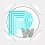## Q. Using the arrays created in Question above,

Write NumPy commands for the following:

a) Use NumPy. split() to split the array myarray2 into 5 arrays columnwise. Store your resulting

arrays in myarray2A, myarray2B, myarray2C, myarray2D and myarray2E. Print the arrays myarray2A, myarray2B, myarray2C, myarray2D and myarray2E.

b) Split the array zeros at array index 2, 5, 7, 8 and store the resulting arrays in zerosA, zerosB, zerosC and zerosD and print them.

c) Concatenate the arrays myarray2A, myarray2B and myarray2C into an array having 3 rows and 3 columns.

(a)

To split the array myarray2 into 5 arrays column-wise using np.split() in NumPy, you can specify the axis parameter as 1 to split along the columns.

```import numpy as np
myarray2 = np.arange(4, 4 + 3 * 5 * 4, 4, dtype=float).reshape(3, 5)
# Split myarray2 into 5 arrays column-wise
myarray2A, myarray2B, myarray2C, myarray2D, myarray2E = np.split(myarray2, 5, axis=1)
print("myarray2A:")
print(myarray2A)
print("\nmyarray2B:")
print(myarray2B)
print("\nmyarray2C:")
print(myarray2C)
print("\nmyarray2D:")
print(myarray2D)
print("\nmyarray2E:")
print(myarray2E)
```

(b)

To split the array zeros at specific indices using np.split() in NumPy, you can provide a list of indices where you want to split the array.

```zeros = np.zeros((10,))

# Split the array zeros at indices 2, 5, 7, 8
zerosA, zerosB, zerosC, zerosD = np.split(zeros, [2, 5, 7, 8])

print("zerosA:")
print(zerosA)
print("\nzerosB:")
print(zerosB)
print("\nzerosC:")
print(zerosC)
print("\nzerosD:")
print(zerosD)
```

(c)

To concatenate arrays myarray2A, myarray2B, and myarray2C into a new array with 3 rows and 3 columns in NumPy, you can use the np.concatenate() function

`concatenated_array = np.concatenate((myarray2A, myarray2B, myarray2C), axis=1)`

You can help us by Clicking on ads. ^_^
Please do not send spam comment : )

1.hii moderator, how are you?,

1.2.#Rushil
Pls trend this hastag